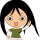Home Communities
IT Knowledge
Inspiration
Languages
EN

# Python - change value of items within specific range

0 points
Created by:Kadeem-Craig
516

In this article, we would like to show you how to change value of items within specific range in Python.

Quick solution:

``````my_list = ['A', 'B', 'C', 'D']

my_list[1:3] = ['X', 'Y']

print(my_list)  # ['A', 'X', 'Y', 'D']``````

## Practical example

Example 1

In this example, we change the values under index `1`-`3` (`A`, `B`) to `X` and `Y`.

``````my_list = ['A', 'B', 'C', 'D']

my_list[1:3] = ['X', 'Y']

print(my_list)  # ['A', 'X', 'Y', 'D']``````

Output:

``['A', 'X', 'Y', 'D']``

Note:

You may change the length of the list by inserting the number of items that doesn't match the number of replaced items.

Example 2

In this example, we change two values under `1`-`3` indexes (`A`, `B`) to the three new values (`X`,`Y`,`Z`). Notice that the length of our array will increase from 4 to 5.

``````my_list = ['A', 'B', 'C', 'D']

my_list[1:3] = ['X', 'Y', 'Z']

print(my_list)  # ['A', 'X', 'Y', 'Z', 'D']``````

Output:

``['A', 'X', 'Y', 'Z', 'D']``

## Alternative titles

Join to our subscribers to be up to date with content, news and offers.